Electron. J. Diff. Eqns., Vol. 2007(2007), No. 100, pp. 1-22.

### A numerical scheme using multi-shockpeakons to compute solutions of the Degasperis-Procesi equation Håkon A. Hoel

Abstract:
We consider a numerical scheme for entropy weak solutions of the DP (Degasperis-Procesi) equation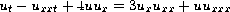. Multi-shockpeakons, functions of the form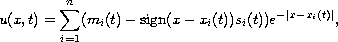are solutions of the DP equation with a special property; their evolution in time is described by a dynamical system of ODEs. This property makes multi-shockpeakons relatively easy to simulate numerically. We prove that if we are given a non-negative initial function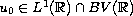such that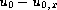is a positive Radon measure, then one can construct a sequence of multi-shockpeakons which converges to the unique entropy weak solution in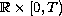for any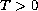. From this convergence result, we construct a multi-shockpeakon based numerical scheme for solving the DP equation.

Submitted June 13, 2007. Published July 19, 2007.
Math Subject Classifications: 35Q53, 37K10.
Key Words: Shallow water equation; numerical scheme; entropy weak solution; shockpeakon; shockpeakon collision.

Show me the PDF file (804 KB), TEX file, and other files for this article.Håkon A. Hoel Centre of Mathematics for Applications, University of Oslo P.O. Box 1053, Blindern, NO-0316 Oslo, Norway email: haakonah1@gmail.com http://www.folk.uio.no/haakonah